# SAT Math Multiple Choice Question 826: Answer and Explanation

### Test Information

Question: 826

1.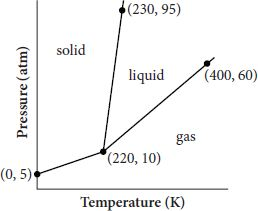A phase diagram shows the temperatures and pressures at which a chemical substanceexists in a certain phase (solid, liquid, or gas). A sample phase diagram for a fictionalsubstance is shown in the figure, where T is temperature in Kelvin and P is pressure in atmospheres. Where on the diagram a certain temperature and pressurecombination falls determines the state(s) in which the substance exists. For example,if a certain temperature-pressure pairing falls in the gas area (but not on the linesegment between gas and liquid), the substance exists only as a gas. However, if thetemperature-pressure pairing is on the line segment between gas and liquid, the substanceexists as both a gas and a liquid. Assuming T > 0 and P > 0, which of the following systems of inequalities could be used to describe thetemperature and pressure ranges in which this substance exists only as a liquid?

• A.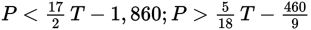• B.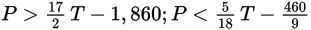• C.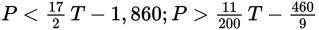• D.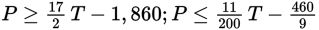Explanation:

A

Difficulty: Hard

Category: Heart of Algebra / Inequalities

Strategic Advice: Sometimes, it is not necessary to apply brute force to a question. Here, you can get away with doing minimal math because the slopes of the lines are enough to answer the question-you do not need to find the y-intercepts.

Getting to the Answer: The question asks for a system of inequalities that describes the region where the substance exists as a liquid only, so you need two restricted (greater/less than but not equal to) inequalities. This means you can eliminate D. Next, examine the lines separating "solid" and "liquid," and "liquid" and "gas." The "liquid" region is below the line that passes through (220, 10) and (230, 95), and it is above the line that passes through (220, 10) and (400, 60). Find the slope of each line:

Below line (<) that has a slope of: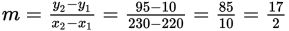above line (>) that has a slope of: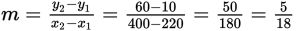Now examine the inequality symbols and the slopes in the remaining answer choices: B is incorrect because the symbols are reversed, and C is incorrect because the slope of the second line is not right. You already eliminated D, so (A) must be the correct answer.